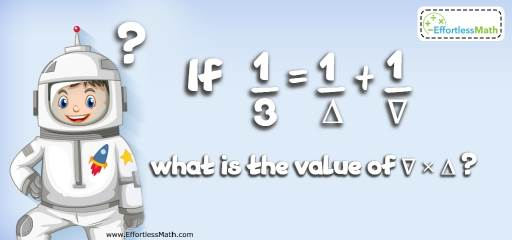# Intelligent Math Puzzle – Challenge 86

This math puzzle does not require any special mathematical knowledge, just logical reasoning, and creative thinking.## Challenge:

If $$\frac{1}{3} = \frac{1}{\triangle} + \frac{1}{\triangledown}$$ what is the value of $$\triangledown × \triangle$$ ?

### The Absolute Best Book to Challenge Your Smart Student!

$$\triangledown$$ and $$\triangle$$ are two digits. The sum of two fractions $$\frac{1}{\triangle}$$ and $$\frac{1}{\triangle}$$ equals to $$\frac{1}{3}$$. Obviously, there are many values for $$\triangledown$$ and $$\triangle$$ that can be used in the equation.
Choose a value for $$\triangledown$$. Let’s put 4 for $$\triangledown$$. Then:
$$\frac{1}{3} = \frac{1}{\triangle} + \frac{1}{4} →\frac{1}{3} – \frac{1}{4} = \frac{1}{\triangle} → \frac{4}{12} – \frac{3}{2} = \frac{1}{\triangle} → \frac{1}{12} = \frac{1}{\triangle} →\triangle = 12$$
Therefore: $$\triangledown × \triangle = 4 × 12 = 48$$

The Best Books to Ace Algebra

### What people say about "Intelligent Math Puzzle – Challenge 86 - Effortless Math: We Help Students Learn to LOVE Mathematics"?

No one replied yet.

X
45% OFF

Limited time only!

Save Over 45%

SAVE $40 It was$89.99 now it is \$49.99